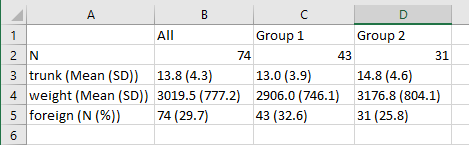# July 2019 update

I have since discovered the excellent table1_mc program and have abandoned using this program. I’m keeping this code here for historical sake. Check out my write-up of table1_mc.

# Stata programs to make tables

Here are a few simple Stata programs that will write a CSV file for your Table 1.

This has three parts:

1. Header (writes the first line of the table with names and the row with Ns),
2. Program for continuous variables, and
3. Program for dichotomous variables.

Each time you want to add another line your table, just call the appropriate program followed by the variable of interest.

```clear all // get rid of everything in memory
webuse auto.dta, clear

set seed 12345
gen treatment = .
replace treatment = round(runiform()) // make a random treatment
// variable that's 0 or 1

// Header + second row with Ns
quietly {
capture log close table1 // force closes any tables with the same name
log using "my_table_1.csv", text replace name(table1) //replace will erase
// any CSV files you started already with the same name
noisily disp ",All,Group 1,Group 2" // line 1
count
local nall=r(N)
count if treatment==0
local ntreatment0=r(N)
count if treatment==1
local ntreatment1=r(N)
noisily disp "N," `nall' "," `ntreatment0' "," `ntreatment1'
log close table1
}

// Program for continuous variables
capture program drop table1_cont // drops any programs with the same name
program define table1_cont
quietly {
syntax varlist
capture log close table1
log using "my_table_1.csv", text append name(table1) // append will
// keep writing onto existing tables
foreach var of varlist `varlist' {
sum `var'
local `var'mean = r(mean)
local `var'sd = r(sd)
local `var'n=r(N)
sum `var' if treatment==0
local `var'mean0 = r(mean)
local `var'sd0 = r(sd)
local `var'n0=r(N)
sum `var' if treatment==1
local `var'mean1 = r(mean)
local `var'sd1 = r(sd)
local `var'n1=r(N)

noisily disp "`var' (Mean (SD))," ///
%3.1f ``var'mean' " (" %3.1f ``var'sd' "),"  ///
%3.1f ``var'mean0' " (" %3.1f ``var'sd0' "),"  ///
%3.1f ``var'mean1' " (" %3.1f ``var'sd1' ")"
} // end varlist loop
log close table1
} // end quietly
end

// program for dichotomous variables
capture program drop table1_dichotomous
program define table1_dichotomous
quietly {
syntax varlist
capture log close table1
log using "my_table_1.csv", text append name(table1)
foreach var of varlist `varlist' {
sum `var'
local `var'n= r(N)
local `var'mean = r(mean)*100
sum `var' if treatment==0
local `var'n0 = r(N)
local `var'mean0 = r(mean)*100
sum `var' if treatment==1
local `var'n1 = r(N)
local `var'mean1 = r(mean)*100
noisily disp "`var' (N (%))," ///
``var'n' " (" %3.1f ``var'mean' "),"  ///
``var'n0' " (" %3.1f ``var'mean0' ")," ///
``var'n1' " (" %3.1f ``var'mean1' ")"
}
log close table1
}
end
// now just call these programs as needed:

table1_cont trunk
table1_cont weight
table1_dichotomous foreign
// and so-on
```

Here is the output from the example above:

,All,Group 1,Group 2
N,74,43,31
trunk (Mean (SD)),13.8 (4.3),13.0 (3.9),14.8 (4.6)
weight (Mean (SD)),3019.5 (777.2),2906.0 (746.1),3176.8 (804.1)
foreign (N (%)),74 (29.7),43 (32.6),31 (25.8)

If opened with MS Excel, it will look like this: